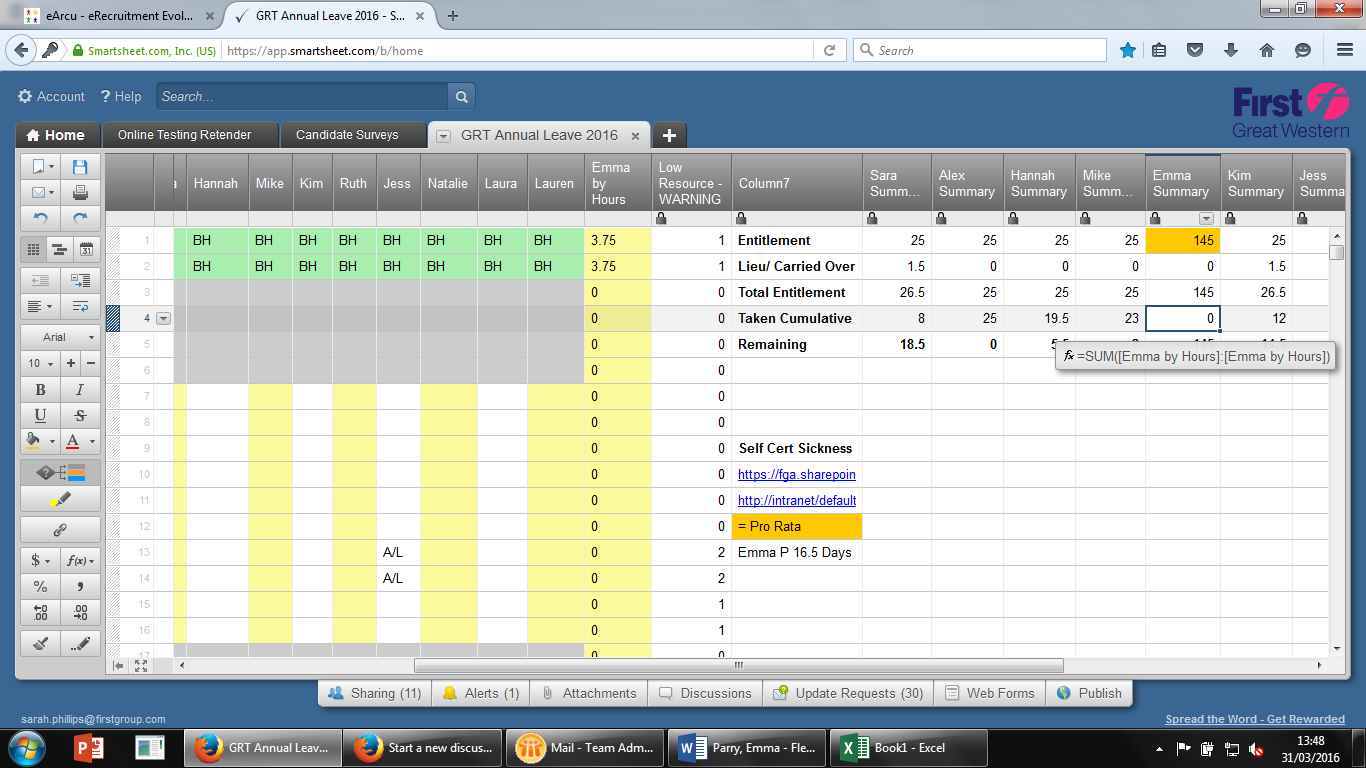#### Welcome to the Smartsheet Forum Archives

The posts in this forum are no longer monitored for accuracy and their content may no longer be current. If there's a discussion here that interests you and you'd like to find (or create) a more current version, please Visit the Current Forums.

# Summing a Column where contents are formula results

Hi

I have a column of numbers which are the results of a formula. I then want to add up the column of numbers but when I use a sum formula it just gives me a blank - can anyone assist?• Are you sure the results are numbers? (Are they alligned to the right automatically?) What formula do you use?

• Hi - sum in column.....=IF(Emma1 = "A/L", "3.75", "0")

Sum adding up results from column in one cell - =SUM([Emma by Hours]:[Emma by Hours])

The column is formatted as text/ number......

• Sara,

3.75 is a number

"3.75" is text.

=IF(Emma1 = "A/L", 3.75, 0)

and see if that helps.

Craig

This discussion has been closed.